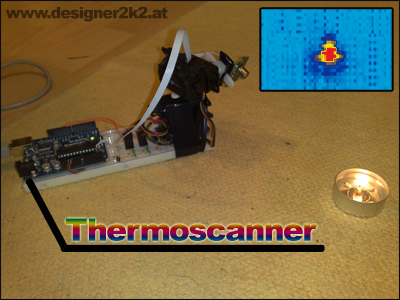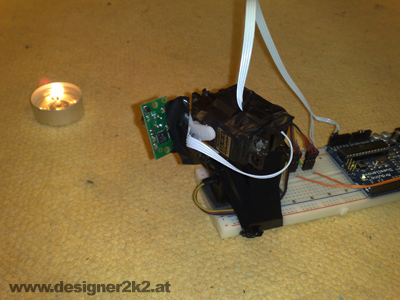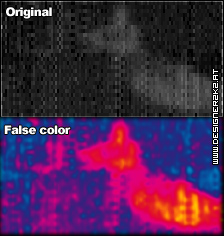# ThermoscannerThermography was never so easy/cheapTake the hardware of the 3d Scanner, and just replacing the distance sensor with a thermopile. Thats it, and there it goes, the Thermoscanner!

## Hardware:

This time im using tape, as i was running out of zip tiesJust combine the 2 RC Servos and stick the TPA81 onto it.

## Theorie:

The TPA81 provides 1x8 Pixels with 5.12° by 6°. The Sensor is mounted verticaly.

To Increase the resolution its stepping 1° horizontal and about 2° verticaly.

This makes is possible to get a real nice resolution, as can be seen in the top picture showing the candle.

The Thermal resolution from the TPA81 is 1°, but its jumping +-1° so measuring a human in a room is tricky...

(Pictrue from me on the couch)

## Software:

The Software is splitted, on part Arduino Code, and the other is Processing.

The Arduino is only listening to the Processing Application, and this is then drawing a nice picture from it.

Processing makes the picture above, below is it processed in false color:This is me on a couch wearing a tshirt and shorts, my face/arms/legs are hotter as they are not covered by clothing.

Taking this picture took about 30seconds with the code provided below.

## Arduino Code:

`//test by d2k2 24.09.2009#include <AFSoftSerial.h>#include <Wire.h>#define TPA81ADDR (0xd0>>1)#include   Servo myServoPan;  // create servo object to control a servo Servo myServoTilt;  // create servo object to control a servo AFSoftSerial mySerial =  AFSoftSerial(3, 2);char CurrentServo;int value;int valueA;          //Storageint valueB;int fresh = 0;int Read = 0;int runVal = 0;void setup()  {  Wire.begin();        // join i2c bus (address optional for master)   myServoPan.attach(9);  // attaches the servo on pin 9 to the servo object   myServoTilt.attach(10);  // attaches the servo on pin 10 to the servo object   pinMode(13, OUTPUT);  Serial.begin(57600);    Serial.println("Scanturm V0.1");}void loop()                     // run over and over again{      get_keyboard_commands();  }void get_keyboard_commands() {  myServoPan.write(valueA);  myServoTilt.write(valueB);      if ( Serial.available()) {        // read in a string     Read = Serial.read();          if (Read == 200){      runVal = 0;     // Serial.println("Reset");       };                     switch (runVal) {    case 0:      runVal = 1;      break;      case 1:      valueA = Read;      runVal = 2;      break;    case 2:      valueB = Read;      runVal = 3;    case 3:         SendTPA();      /*      Serial.print(": ");      Serial.print(val);        Serial.println("\$");      */      //runVal = 1;      break;   }  }  }void SendTPA(){    //Send the TPA81 Data back to the PC:     byte b;  int i;   for (i=1; i<=9; i++)  {    Wire.beginTransmission(TPA81ADDR);    Wire.send(i);    Wire.endTransmission();        Wire.requestFrom(TPA81ADDR, (int) 1);    while(Wire.available() < 1)   { ; }    b = Wire.receive(); // receive a byte as character     Serial.print(b, DEC);    Serial.print(";");  }  Serial.println("");}`

## Processing Code:

`//d2k2 24.09.2009  shows values from the TPA81 //prestage for putting the sensor on the scan turrentimport processing.serial.*;Serial myPort;  // Create object from Serial classint lf = 10;    // Linefeed in ASCIIString myString;int[] val = {1,1,1,1,1,1,1,1,1};int MaxT=100;int MinT=20;int pan = 40;int tilt = 80;String[] list = null;int MaxT2 = 0;int MinT2 = 255;boolean result = false;boolean run = false;long time;long lastrun = 0;long runT;    void setup() {  size(400, 400);  String portName = Serial.list();  myPort = new Serial(this, portName, 57600);  myPort.buffer(1);}void draw(){    time = millis();  runT =  lastrun - time;  String myTest = "";   if ( myPort.available() > 0) {  // If data is available,       myString = myPort.readStringUntil(lf);    if (myString != null)     {      list = split(myString, ';');      myTest = list;        if(int(myTest)>0)      {        result = true;                val = int(list);        val = int(list);        val = int(list);        val = int(list);        val = int(list);        val = int(list);        val = int(list);        val = int(list);        val = int(list);                //check for new max/min events                if(MaxT2<max(val))        {          MaxT2 = max(val);        }                if(MinT2>min(val))        {          MinT2 = min(val);        }                         //Draw another Line, right at the Pan Position         //Pan is about everywhere from 10 to 170         //so i need to update every Pan Pixel and just try it like that now                  float Col = 0;         for(int i = 1;i<9; i = i+1){           Col = map(val[i],MinT,MaxT,0,255);           color drp = color(Col,Col,Col);           for(int i2 = 1; i2 < 5; i2 = i2+1){           set(pan+pan,    (i*4)+75+i2+tilt+tilt+4*i-100,drp);           }           for(int i2 = 1; i2 < 5; i2 = i2+1){             set(pan+1+pan,(i*4)+75+i2+tilt+tilt+4*i-100,drp);           }         }                              }  }  //background(255);             // Set background to white//Draw them 9 Pixels in a line:/*int Size = 45;         float Col = 0;        for (int i = 1; i < 9; i = i+1) {           rect((Size*i)-Size+5, 5, Size, Size);           Col = map(val[i],MinT,MaxT,0,255);           fill(0,0,Col);        }*/  }if(millis()>lastrun){if(run == true){  //Now i need to check if i got a result:  if(result == true)  {    //Now i need to check if im at the end of my motion:    if(pan < 150)    {      //Now i can move :)      pan = pan + 1;      SetServo();      lastrun = millis()+100;    }     else    {      run = false;    }  }}}    }void keyPressed() {  if( key == '6'){   pan = pan+1;     SetServo();   println("Pan: " + pan);      println("Tilt: " + tilt);     }    if( key == '4'){   pan = pan-1;   SetServo();   println("Pan: " + pan);      println("Tilt: " + tilt);     }   if( key == '8'){   tilt = tilt-2;   SetServo();   println("Pan: " + pan);      println("Tilt: " + tilt);     }    if( key == '2'){   tilt = tilt+2;   SetServo();   println("Pan: " + pan);      println("Tilt: " + tilt);     }    if( key == '5'){   SetServo();   println("Pan: " + pan);      println("Tilt: " + tilt);     }    if( key == '1'){   pan = 40;   SetServo();   println("Pan: " + pan);      println("Tilt: " + tilt);     }    if( key == '3'){   run = true;  } }void SetServo(){    result = false;    myPort.write(200); //init    myPort.write(tilt); //tilt    myPort.write(pan); //pan    delay(100);    myPort.write(80); //trigger read     }`

`Arduino Forum: http://www.arduino.cc/cgi-bin/yabb2/YaBB.pl?num=1253898939/0#0Hack a Day: http://hackaday.com/2009/09/25/arduino-thermoscanner/Make: http://blog.makezine.com/archive/2009/09/thermal_imaging_on_the_cheap.html`